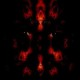# Gematria

## Esoterica : Codexian Trinkets

Each of the following numbersets have been systematically calculated to prove that certain synchronistic parallels and connections exist between specific, particularly esoteric subjects. Intelligent ‘Reality’.

732 = ( M U S H R O O M )

732 = On The Trail (Or Trial)
732 = Training Day
732 = Save The Self
732 = The Forsaken
732 = You + You
732 = King Of Kings
732 = God In Number
732 = Eat A God’s Flesh
732 = Mushroom
732 = I Am The Myth
732 = The Religion
732 = A Funny Joke
732 = A First Clone
732 = Satan’s Son
732 = He Is The Son
732 = God Called Me Jesus
732 = Neon Jesus
732 = He Ate The Apple
732 = Fruit Tree
732 = A Perfect Tree
732 = A Number Tree
732 = The Shaman Seed
732 = Spirit + GATC (Genome Letters)
732 = The Magic Poet
732 = The Good Evil
732 = The Dark Light
732 = The Magic Light
732 = A Revelation

4 4 4 = ( G E M A T R I A )

444 = The Lock
444 = The Key
444 = Jesus
444 = Lucifer
444 = Fire And Ice
444 = Male + Female
444 = A Union
444 = Connect
444 = A Number

_______________________________________
( G e m a t r i a – K e y )
A = 6 | B = 12 | C = 18 |-(…)-| X = 144 | Y = 150 | Z = 156

( M I S C E L L A N E O U S )

156 =
God
Data
Z (Alphabet)
Qi
Gen (root: to create)
Dog
Mega
A Gem
Bio
Mage
Eat
Ate
Dial
Heal
Lie

168 =
Jedi
Man
Is
Child
Lamb
We
Need
Eden
A Bud
Jr.
A Dance
A Dick
A Code
Dare
A Bet

198:
A Life
A File
A Cell
Ice Cage
A Coma
Find
Phi
The
Mean
Magic
Seed
Herb
Ganja
A High
Gate
Or
Name
A Pig
A Bomb
Amen

## 0 thoughts on “Gematria”

1.omnimage says:

Create new wordpress blog for gematria ?